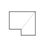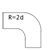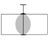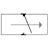Calculating Pressure Drop

Please use a decimal point and not a comma to indicate the decimal place!

The green rows indicated contain results.

The calculation is valid for smooth pipes.

• SI - unit
• US - unit
• Flow rate
V
m³/min
Nominal pipe length
L
m
Nominal pipe inside diameter
Id
mm
Working pressure absolute
p
bar
Pressure drop
Δp
bar

The nominal length of the pipework is calculated by adding the straight pipe runs and the equivalent length of each joint or fitting.

The equivalent lengths of the joints and fittings can be calculated from the list or simply estimated as 60% of the straight runs, i.e. total length = total straight runs x 1.6.

Calculation Equivalent pipe length
• SI - unit
• US - unit
• Pipe diameter
DN
equivalent length[m]
Number
Elbow1.5
Elbow R=2d0.3
Elbow R=d0.4
T-piece through0.5
T-piece branch1.5
Seated valve5
Butterfly / ball valve0.3
Check valve2
Reduction piece (DN): 200.5
Equivalent lengths of joints and fittings:
m